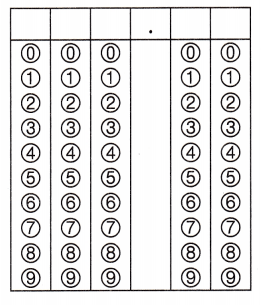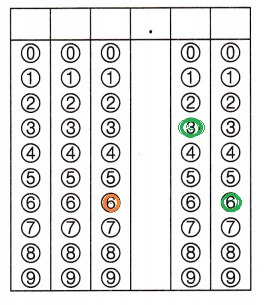Refer to our Texas Go Math Grade 5 Answer Key Pdf to score good marks in the exams. Test yourself by practicing the problems from Texas Go Math Grade 5 Module 10 Assessment Answer Key.

Vocabulary

Choose the best term from the box.

Vocabulary
input/output table
pattern
pattern rule

Question 1.
You can use a graph or an __________ to show a pattern. (p. 375)
pattern rule,

Explanation:
We will use a graph or pattern rule to show a pattern,
Pattern rules means numerical pattern is a sequence of numbers
that has been created based on a formula or rule called a pattern rule.
Pattern rules can use one or more mathematical operations to describe
the relationship between consecutive numbers in the pattern.

Question 2.
A __________ is an ordered set of numbers or objects. (p. 375)
Pattern,

Explanation:
Pattern means an ordered set of numbers or objects in which the
order helps you predict what will come next.

Use the rule to complete the table. (TEKS 5.4.C)Concepts and Skills

Question 3.
Rule: m = n + 10.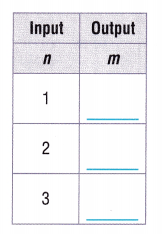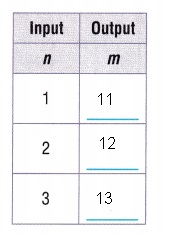Explanation:
Given Rule m = n + 10,
if n = 1 then m = 1 + 10 = 11, (1,11),
if  n = 2 then m = 2 + 10 = 12, (2,12),
if n= 3 then m = 3 + 10 = 13, (3,13) as shown
above in the table.

Question 4.
Rule: p = s × 4.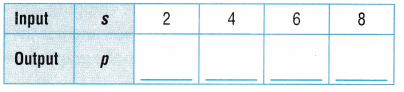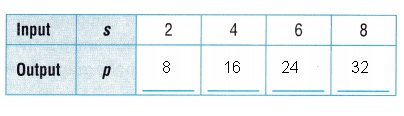Explanation:
Given p = s X  4,
if s = 2 then p = 2 X 4 = 8, (2,8),
if s = 4 then p = 4 X 4 = 16, (4,16),
if s = 6 then p = 6 X 4 = 24, (6, 24),
if s = 8 then p = 8 X 4 = 32,(8,32) as shown
above in the table.

Decide if the pattern shown in the table is additive or multiplicative.
Write a rule to describe the pattern. (TEKS 5.4.D)

Question 5.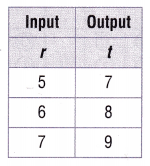The pattern is ____________.
Rule: ____________
Rule is t = r + 2,

Explanation:
As we see if input r = 5 then output t = 5 + 2 = 7,
if input r = 6 then output t = 6 + 2 = 8 and
if input r = 7 then output t = 7 + 2 = 9 therefore
the pattern is additive and rule is t = r + 2.

Question 6.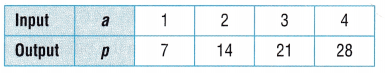The pattern is ____________.
Rule: ____________
The pattern is multiplicative,
Rule: p = a X 7,

Explanation:
As we see if input a = 1 then output p = 1 X 7 = 7,
if input a = 2 then output p = 2 X 7= 14
if input a = 3 then output p = 3 X 7 = 21 and
if input a = 4 then output p = 4 X 7 = 28 therefore
the pattern is multiplicative and rule is p = a x 7.

Use the graph for 7-8.Question 7.
The graph shows the relationship between the number of
work days and the number of weeks. Which rule best describes the
pattern in the graph? (TEKS 5.4.D)
(A) d = w + 5
(B) w = 5d
(C) d = 5w
(D) w = d + 5
(B) w = 5d,

Explanation:
The graph shows the relationship between the number of
work days and the number of weeks. the rule best describes the
pattern in the graph is w = 5d as if d = 1 then w = 5 X  1 = 5,
d = 2 then w = 5 X 2 = 10,
if d = 3 then w = 5 X 3 = 15,
if d = 4 then w = 5 X 4 = 20 so the rule is w = 5d
which matches with (B).

Question 8.
Which of the following number pairs extends the pattern on the graph? (TEKS 5.4.C)
(A) (5, 25)
(B) (6, 25)
(C) (25, 5)
(D) (5, 20)
(A) (5, 25),

Explanation:
Number pairs extends the pattern on the graph is as w = 5d after d = 4
the next one is d = 5 so w = 5 x 5 = 25 therefore next number pair
extends the pattern on the graph is (5,25) matches with (A).

Question 9.
Party favors cost $1, plus$5 for shipping. The rule is t = 5 + p,
where t is the total cost in dollars and p is the number of party favors bought.
Which point on the graph shows the total cost of 4 party favors? (TEKS 5.4.C)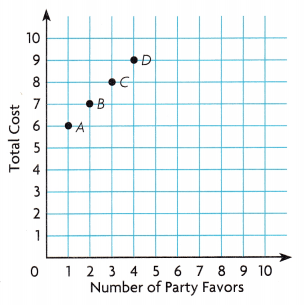(A) Point A
(B) Point B
(C) Point C
(D) Point D
(D) Point D

Explanation:
Given Party favors cost $1, plus$5 for shipping. The rule is t = 5 + p,
where t is the total cost in dollars and p is the number of party favors bought.
the point on the graph shows the total cost of 4 party favors is if p = 4
then t = 5 + 4 = 9 so point D, (4,9) matches with (D).

Question 10.
The rule for a pattern is e = 6w. What is the output, e, when the input, w, is 6? (TEKS 5.4.C)